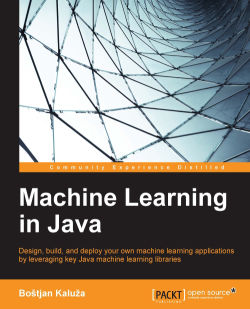### Machine Learning in Java4.8 (8 reviews total)
By Bostjan Kaluza

• Constantly updated with 100+ new titles each month
• Breadth and depth in over 1,000+ technologies

As the amount of data continues to grow at an almost incomprehensible rate, being able to understand and process data is becoming a key differentiator for competitive organizations. Machine learning applications are everywhere, from self-driving cars, spam detection, document search, and trading strategies, to speech recognition. This makes machine learning well-suited to the present-day era of Big Data and Data Science. The main challenge is how to transform data into actionable knowledge.

Machine Learning in Java will provide you with the techniques and tools you need to quickly gain insight from complex data. You will start by learning how to apply machine learning methods to a variety of common tasks including classification, prediction, forecasting, market basket analysis, and clustering.

Moving on, you will discover how to detect anomalies and fraud, and ways to perform activity recognition, image recognition, and text analysis. By the end of the book, you will explore related web resources and technologies that will help you take your learning to the next level.

By applying the most effective machine learning methods to real-world problems, you will gain hands-on experience that will transform the way you think about data.

Publication date:
April 2016
Publisher
Packt
Pages
258
ISBN
9781784396589

## Chapter 1. Applied Machine Learning Quick Start

This chapter introduces the basics of machine learning, laying down the common themes and concepts and making it easy to follow the logic and familiarize yourself with the topic. The goal is to quickly learn the step-by-step process of applied machine learning and grasp the main machine learning principles. In this chapter, we will cover the following topics:

• Introducing machine learning and its relation to data science

• Discussing the basic steps in applied machine learning

• Discussing the kind of data we are dealing with and its importance

• Discussing approaches of collecting and preprocessing the data

• Making sense of data using machine learning

• Using machine learning to extract insights from data and build predictors

If you are already familiar with machine learning and are eager to start coding, then quickly jump to the following chapters. However, if you need to refresh your memory or clarify some concepts, then it is strongly recommend to revisit the topics presented in this chapter.

## Machine learning and data science

Nowadays, everyone talks about machine learning and data science. So, what exactly is machine learning anyway? How does it relate to data science? These two terms are commonly confused, as they often employ the same methods and overlap significantly. Therefore, let's first clarify what they are.

Josh Wills tweeted:

 "Data scientist is a person who is better at statistics than any software engineer and better at software engineering than any statistician". --(Josh Wills)

More specifically, data science encompasses the entire process of obtaining knowledge from data by integrating methods from statistics, computer science, and other fields to gain insight from data. In practice, data science encompasses an iterative process of data harvesting, cleaning, analysis and visualization, and deployment.

Machine learning, on the other hand, is mainly concerned with fairly generic algorithms and techniques that are used in analysis and modeling phases of data science process. Arthur Samuel proposed the following definition back in 1995:

 "Machine Learning relates with the study, design and development of the algorithms that give computers the capability to learn without being explicitly programmed." --Arthur Samuel

### What kind of problems can machine learning solve?

Among the different machine learning approaches, there are three main ways of learning, as shown in the following list:

• Supervised learning

• Unsupervised learning

• Reinforcement learning

Given a set of example inputs, X, and their outcomes, Y, supervised learning aims to learn a general mapping function, f, that transforms inputs to outputs, as f: X Y

An example of supervised learning is credit card fraud detection, where the learning algorithm is presented with credit card transactions (matrix X) marked as normal or suspicious. (vector Y). The learning algorithm produces a decision model that marks unseen transactions as normal or suspicious (that is the f function).

In contrast, unsupervised learning algorithms do not assume given outcome labels, Y as they focus on learning the structure of the data, such as grouping similar inputs into clusters. Unsupervised learning can, hence, discover hidden patterns in the data. An example of unsupervised learning is an item-based recommendation system, where the learning algorithm discovers similar items bought together, for example, people who bought book A also bought book B.

Reinforcement learning addresses the learning process from a completely different angle. It assumes that an agent, which can be a robot, bot, or computer program, interacts with a dynamic environment to achieve a specific goal. The environment is described with a set of states and the agent can take different actions to move from one state to another. Some states are marked as goal states and if the agent achieves this state, it receives a large reward. In other states, the reward is smaller, non-existing, or even negative. The goal of reinforcement learning is to find an optimal policy, that is, a mapping function that specifies the action to take in each of the states without a teacher explicitly telling whether this leads to the goal state or not. An example of reinforcement learning is a program for driving a vehicle, where the states correspond to the driving conditions—for example, current speed, road segment information, surrounding traffic, speed limits, and obstacles on the road—and the actions can be driving maneuvers such as turn left or right, stop, accelerate, and continue. The learning algorithm produces a policy that specifies the action that is to be taken in specific configuration of driving conditions.

In this book, we will focus on supervised and unsupervised learning only, as they share many concepts. If reinforcement learning sparked your interest, a good book to start with is Reinforcement Learning: An Introduction by Richard S. Sutton and Andrew Barto.

### Applied machine learning workflow

This book's emphasis is on applied machine learning. We want to provide you with the practical skills needed to get learning algorithms to work in different settings. Instead of math and theory of machine learning, we will spend more time on the practical, hands-on skills (and dirty tricks) to get this stuff to work well on an application. We will focus on supervised and unsupervised machine learning and cover the essential steps from data science to build the applied machine learning workflow.

A typical workflow in applied machine learning applications consists of answering a series of questions that can be summarized in the following five steps:

1. Data and problem definition: The first step is to ask interesting questions. What is the problem you are trying solve? Why is it important? Which format of result answers your question? Is this a simple yes/no answer? Do you need to pick one of the available questions?

2. Data collection: Once you have a problem to tackle, you will need the data. Ask yourself what kind of data will help you answer the question. Can you get the data from the available sources? Will you have to combine multiple sources? Do you have to generate the data? Are there any sampling biases? How much data will be required?

3. Data preprocessing: The first data preprocessing task is data cleaning. For example, filling missing values, smoothing noisy data, removing outliers, and resolving consistencies. This is usually followed by integration of multiple data sources and data transformation to a specific range (normalization), to value bins (discretized intervals), and to reduce the number of dimensions.

4. Data analysis and modeling with unsupervised and supervised learning: Data analysis and modeling includes unsupervised and supervised machine learning, statistical inference, and prediction. A wide variety of machine learning algorithms are available, including k-nearest neighbors, naïve Bayes, decision trees, support vector machines, logistic regression, k-means, and so on. The choice of method to be deployed depends on the problem definition discussed in the first step and the type of collected data. The final product of this step is a model inferred from the data.

5. Evaluation: The last step is devoted to model assessment. The main issue models built with machine learning face is how well they model the underlying data—if a model is too specific, that is, it overfits to the data used for training, it is quite possible that it will not perform well on a new data. The model can be too generic, meaning that it underfits the training data. For example, when asked how the weather is in California, it always answers sunny, which is indeed correct most of the time. However, such a model is not really useful for making valid predictions. The goal of this step is to correctly evaluate the model and make sure it will work on new data as well. Evaluation methods include separate test and train set, cross-validation, and leave-one-out validation.

In the following sections, we will take a closer look at each of the steps. We will try to understand the type of questions we must answer during the applied machine learning workflow and also look at the accompanying concepts of data analysis and evaluation.

## Data and problem definition

Data is simply a collection of measurements in the form of numbers, words, measurements, observations, descriptions of things, images, and so on.

### Measurement scales

The most common way to represent the data is using a set of attribute-value pairs. Consider the following example:

```Bob = {
height: 185cm,
eye color: blue,
hobbies: climbing, sky diving
}```

For example, `Bob` has attributes named `height`, `eye color`, and `hobbies` with values `185cm`, `blue`, `climbing`, `sky diving`, respectively.

A set of data can be simply presented as a table, where columns correspond to attributes or features and rows correspond to particular data examples or instances. In supervised machine learning, the attribute whose value we want to predict the outcome, Y, from the values of the other attributes, X, is denoted as class or the target variable, as follows:

Name

Height [cm]

Eye color

Hobbies

Bob

185.0

Blue

Climbing, sky diving

Anna

163.0

Brown

The first thing we notice is how varying the attribute values are. For instance, height is a number, eye color is text, and hobbies are a list. To gain a better understanding of the value types, let's take a closer look at the different types of data or measurement scales. Stevens (1946) defined the following four scales with increasingly more expressive properties:

• Nominal data are mutually exclusive, but not ordered. Their examples include eye color, martial status, type of car owned, and so on.

• Ordinal data correspond to categories where order matters, but not the difference between the values, such as pain level, student letter grade, service quality rating, IMDB movie rating, and so on.

• Interval data where the difference between two values is meaningful, but there is no concept of zero. For instance, standardized exam score, temperature in Fahrenheit, and so on.

• Ratio data has all the properties of an interval variable and also a clear definition of zero; when the variable equals to zero, there is none of this variable. Variables such as height, age, stock price, and weekly food spending are ratio variables.

Why should we care about measurement scales? Well, machine learning heavily depends on the statistical properties of the data; hence, we should be aware of the limitations each data type possesses. Some machine learning algorithms can only be applied to a subset of measurement scales.

The following table summarizes the main operations and statistics properties for each of the measurement types:

Property

Nominal

Ordinal

Interval

Ratio

Frequency of distribution

Mode and median

Order of values is known

Can quantify difference between each value

Can multiply and divide values

Has true zero

Furthermore, nominal and ordinal data correspond to discrete values, while interval and ratio data can correspond to continuous values as well. In supervised learning, the measurement scale of the attribute values that we want to predict dictates the kind of machine algorithm that can be used. For instance, predicting discrete values from a limited list is called classification and can be achieved using decision trees; while predicting continuous values is called regression, which can be achieved using model trees.

## Data collection

So, where does the data come from? We have two choices: observe the data from existing sources or generate the data via surveys, simulations, and experiments. Let's take a closer look at both the approaches.

### Find or observe data

Data can be found or observed at many places. An obvious data source is the Internet. Intel (2013) presented the following iconographic, showing the massive amount of data collected by different Internet services. In 2013, digital devices created four zettabytes (1021 = billion terabytes) of data. In 2017, it is expected that the number of connected devices will reach three times the number of people on earth; hence, the amount of data generated and collected will increase even further: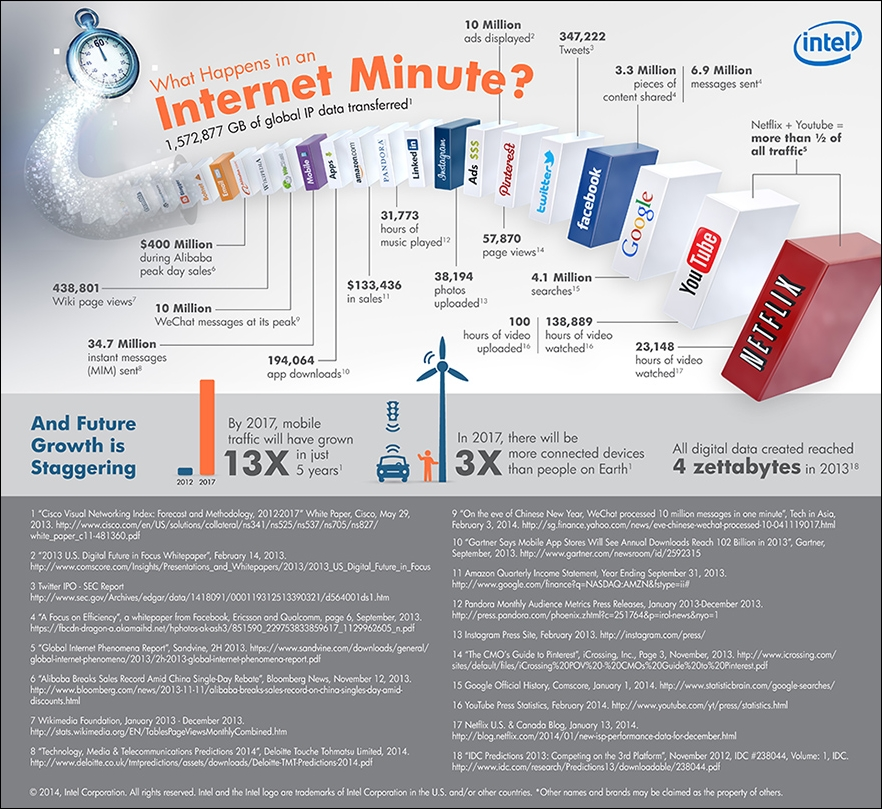To get the data from the Internet, there are multiple options, as shown in the following:

• Bulk downloads from websites such as Wikipedia, IMDb, and Million Song database.

• Web scraping—It is OK to scrape public, non-sensitive, and anonymized data. Be sure to check terms of conditions and to fully reference information.

The main drawbacks of found data are that it takes time and space to accumulate the data; they cover only what happened, for instance, intentions, motivations, or internal motivations are not collected. Finally, such data might be noisy, incomplete, inconsistent, and may even change over time.

Another option is to collect measurements from sensors such as inertial and location sensors in mobile devices, environmental sensors, and software agents monitoring key performance indicators.

### Generate data

An alternative approach is to generate the data by yourself, for example, with a survey. In survey design, we have to pay attention to data sampling, that is, who are the respondents answering the survey. We only get data from the respondents who are accessible and willing to respond. Also, respondents can provide answers that are in line with their self-image and researcher's expectations.

Next, the data can be collected with simulations, where a domain expert specifies behavior model of users at a micro level. For instance, crowd simulation requires specifying how different types of users will behave in crowd, for example, following the crowd, looking for an escape, and so on. The simulation can be then run under different conditions to see what happens (Tsai et al. 2011). Simulations are appropriate for studying macro phenomena and emergent behavior; however, they are typically hard to validate empirically.

Furthermore, you can design experiments to thoroughly cover all the possible outcomes, where you keep all the variables constant and only manipulate one variable at a time. This is the most costly approach, but usually provides the best quality of data.

### Sampling traps

Data collection may involve many traps. To demonstrate one, let me share a story. There is supposed to be a global, unwritten rule for sending regular mail between students for free. If you write student to student to the place where the stamp should be, the mail is delivered to the recipient for free. Now, suppose Jacob sends a set of postcards to Emma, and given that Emma indeed receives some of the postcards, she concludes that all the postcards are delivered and that the rule indeed holds true. Emma reasons that as she received the postcards, all the postcards are delivered. However, she does not possess the information about the postcards that were sent by Jacob, but were undelivered; hence, she is unable to account this into her inference. What Emma experienced is survivorship bias, that is, she drew the conclusion based on the survived data only. For your information, the postcards that are being sent with student to student stamp get a circled black letter T stamp on them, which means postage is due and that receiver should pay it, including a small fine. However, mail services often have higher costs on applying such fee and hence do not do it (Magalhães, 2010).

Another example is a study, which found that the profession with the lowest average age of death was student. Being a student does not cause you to die at an early age, being a student means you are young. This is what makes the average of those that die so low (Gelman and Nolan, 2002).

Furthermore, a study that found that only 1.5% of drivers in accidents reported they were using a cell phone, whereas 10.9% reported another occupant in the car distracted them. Can we conclude that using a cell phone is safer than speaking with another occupant (Uts, 2003)? To answer this question, we need to know the prevalence of the cell phone use. It is likely that a higher number of people talked to another occupant in the car while driving than talking on the cell during the period when the data was collected.

## Data pre-processing

The goal of data pre-processing tasks is to prepare the data for a machine learning algorithm in the best possible way as not all algorithms are capable of addressing issues with missing data, extra attributes, or denormalized values.

### Data cleaning

Data cleaning, also known as data cleansing or data scrubbing, is the process of the following:

• Identifying inaccurate, incomplete, irrelevant, or corrupted data to remove it from further processing

• Parsing data, extracting information of interest, or validating whether a string of data is in an acceptable format

• Transforming data into a common encoding format, for example, utf-8 or int32, time scale, or normalized range

• Transforming data into a common data schema, for instance, if we collect temperature measurements from different types of sensors, we might want them to have the same structure

Now, let's look at some more concrete pre-processing steps.

### Fill missing values

Machine learning algorithms generally do not work well with missing values. Rare exceptions include decision trees, naïve Bayes classifier, and some rule-based learners. It is very important to understand why a value is missing. It can be missing due to many reasons such as random error, systematic error, and sensor noise. Once we identified the reason, there are multiple ways to deal with the missing values, as shown in the following list:

• Remove the instance: If there is enough data, and only a couple of non-relevant instances have some missing values, then it is safe to remove these instances.

• Remove the attribute: Removing an attribute makes sense when most of the values are missing, values are constant, or attribute is strongly correlated with another attribute.

• Assign a special value N/A: Sometimes a value is missing due to valid reasons such as the value is out of scope discrete attribute value is not defined, or it is not possible to obtain or measure the value, which can be an indicator as well. For example, a person never rates a movie, so his rating on this movie is nonexistent.

• Take the average attribute value: In case we have a limited number of instances, we might not be able to afford removing instances or attributes. In that case, we can estimate the missing values, for example, by assigning the average attribute value or the average value over similar instances.

• Predict the value from other attributes: Predict the value from the previous entries if the attribute possesses time dependencies.

As we have seen, the value can be missing for many reasons, and hence, it is important to understand why the value is missing, absent, or corrupted.

### Remove outliers

Outliers in data are values that are unlike any other values in the series and affect all learning methods to various degrees. These can be extreme values, which could be detected with confidence intervals and removed by threshold. The best approach is to visualize the data and inspect the visualization to detect irregularities. An example is shown in the following diagram. Visualization applies to low-dimensional data only: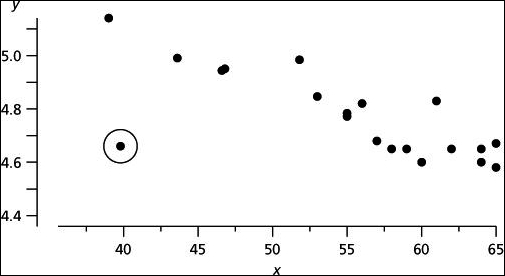### Data transformation

Data transformation techniques tame the dataset to a format that a machine learning algorithm expects as an input, and may even help the algorithm to learn faster and achieve better performance. Standardization, for instance, assumes that data follows Gaussian distribution and transforms the values in such a way that the mean value is zero and the deviation is 1, as follows: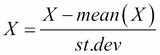Normalization, on the other hand, scales the values of attributes to a small, specified range, usually between 0 and 1: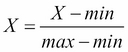Many machine learning toolboxes automatically normalize and standardize the data for you.

The last transformation technique is discretization, which divides the range of a continuous attribute into intervals. Why should we care? Some algorithms, such as decision trees and naïve Bayes prefer discrete attributes. The most common ways to select the intervals are shown in the following:

• Equal width: The interval of continuous variable is divided into k equal-width intervals

• Equal frequency: Suppose there are N instances, each of the k intervals contains approximately N/k instances

• Min entropy: The approach recursively splits the intervals until the entropy, which measures disorder, decreases more than the entropy increase, introduced by the interval split (Fayyad and Irani, 1993)

The first two methods require us to specify the number of intervals, while the last method sets the number of intervals automatically; however, it requires the class variable, which means, it won't work for unsupervised machine learning tasks.

### Data reduction

Data reduction deals with abundant attributes and instances. The number of attributes corresponds to the number of dimensions in our dataset. Dimensions with low prediction power do not only contribute very little to the overall model, but also cause a lot of harm. For instance, an attribute with random values can introduce some random patterns that will be picked up by a machine learning algorithm.

To deal with this problem, the first set of techniques removes such attributes, or in other words, selects the most promising ones. This process is knows as feature selection or attribute selection and includes methods such as ReliefF, information gain, and Gini index. These methods are mainly focused on discrete attributes.

Another set of tools, focused on continuous attributes, transforms the dataset from the original dimensions into a lower-dimensional space. For example, if we have a set of points in three-dimensional space, we can make a projection into a two-dimensional space. Some information is lost, but in case the third dimension is irrelevant, we don't lose much as the data structure and relationships are almost perfectly preserved. This can be performed by the following methods:

• Singular value decomposition (SVD)

• Principal Component Analysis (PCA)

• Neural nets auto encoders

The second problem in data reduction is related to too many instances; for example, they can be duplicates or coming from a very frequent data stream. The main idea is to select a subset of instances in such a way that distribution of the selected data still resembles the original data distribution, and more importantly, the observed process. Techniques to reduce the number of instances involve random data sampling, stratification, and others. Once the data is prepared, we can start with the data analysis and modeling.

## Unsupervised learning

Unsupervised learning is about analyzing the data and discovering hidden structures in unlabeled data. As no notion of the right labels is given, there is also no error measure to evaluate a learned model; however, unsupervised learning is an extremely powerful tool. Have you ever wondered how Amazon can predict what books you'll like? How Netflix knows what you want to watch before you do? The answer can be found in unsupervised learning. The following is one such example.

### Find similar items

Many problems can be formulated as finding similar sets of elements, for example, customers who purchased similar products, web pages with similar content, images with similar objects, users who visited similar websites, and so on.

Two items are considered similar if they are a small distance apart. The main questions are how each item is represented and how is the distance between the items defined. There are two main classes of distance measures: Euclidean distances and non-Euclidean distances.

#### Euclidean distances

In the Euclidean space, with the n dimension, the distance between two elements is based on the locations of the elements in such a space, which is expressed as p-norm distance. Two commonly used distance measures are L2 and L1 norm distances.

L2 norm, also known as Euclidean distance, is the most frequently applied distance measure that measures how far apart two items in a two-dimensional space are. It is calculated as a square root of the sum of the squares of the differences between elements a and b in each dimension, as follows: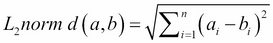L1 norm, also known as Manhattan distance, city block distance, and taxicab norm, simply sums the absolute differences in each dimension, as follows: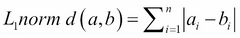#### Non-Euclidean distances

A non-Euclidean distance is based on the properties of the elements, but not on their location in space. Some well-known distances are Jaccard distance, cosine distance, edit distance, and Hamming distance.

Jaccard distance is used to compute the distance between two sets. First, we compute the Jaccard similarity of two sets as the size of their intersection divided by the size of their union, as follows: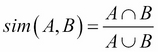The Jaccard distance is then defined as 1 minus Jaccard similarity, as shown in the following: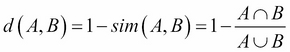Cosine distance between two vectors focuses on the orientation and not magnitude, therefore, two vectors with the same orientation have cosine similarity 1, while two perpendicular vectors have cosine similarity 0. Suppose we have two multidimensional points, think of a point as a vector from origin (0,0, …, 0) to its location. Two vectors make an angle, whose cosine distance is a normalized dot-product of the vectors, as follows: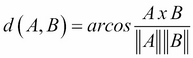Cosine distance is commonly used in a high-dimensional feature space; for instance, in text mining, where a text document represents an instance, features that correspond to different words, and their values corresponds to the number of times the word appears in the document. By computing cosine similarity, we can measure how likely two documents match in describing similar content.

Edit distance makes sense when we compare two strings. The distance between the a=a1a2a3…an and b=b1b2b3…bn strings is the smallest number of the insert/delete operation of single characters required to convert the string from a to b. For example, a = abcd and b = abbd. To convert a to b, we have to delete the second b and insert c in its place. No smallest number of operations would convert a to b, thus the distance is d(a, b)=2.

Hamming distance compares two vectors of the same size and counts the number of dimensions in which they differ. In other words, it measures the number of substitutions required to convert one vector into another.

There are many distance measures focusing on various properties, for instance, correlation measures the linear relationship between two elements: Mahalanobis distance that measures the distance between a point and distribution of other points and SimRank, which is based on graph theory, measures similarity of the structure in which elements occur, and so on. As you can already imagine selecting and designing the right similarity measure for your problem is more than half of the battle. An impressive overview and evaluation of similarity measures is collected in Chapter 2, Similarity and Dissimilarity Measures in the book Image Registration: Principles, Tools and Methods by A. A. Goshtasby (2012).

#### The curse of dimensionality

The curse of dimensionality refers to a situation where we have a large number of features, often hundreds or thousands, which lead to an extremely large space with sparse data and, consequently, to distance anomalies. For instance, in high dimensions, almost all pairs of points are equally distant from each other; in fact, almost all the pairs have distance close to the average distance. Another manifestation of the curse is that any two vectors are almost orthogonal, which means all the angles are close to 90 degrees. This practically makes any distance measure useless.

A cure for the curse of dimensionality might be found in one of the data reduction techniques, where we want to reduce the number of features; for instance, we can run a feature selection algorithm such as ReliefF or feature extraction/reduction algorithm such as PCA.

### Clustering

Clustering is a technique for grouping similar instances into clusters according to some distance measure. The main idea is to put instances that are similar (that is, close to each other) into the same cluster, while keeping the dissimilar points (that is, the ones further apart from each other) in different clusters. An example of how clusters might look is shown in the following diagram: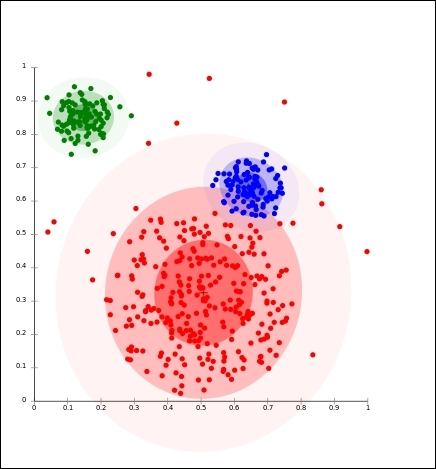The clustering algorithms follow two fundamentally different approaches. The first is a hierarchical or agglomerative approach that first considers each point as its own cluster, and then iteratively merges the most similar clusters together. It stops when further merging reaches a predefined number of clusters or if the clusters to be merged are spread over a large region.

The other approach is based on point assignment. First, initial cluster centers (that is, centroids) are estimated—for instance, randomly—and then, each point is assigned to the closest cluster, until all the points are assigned. The most well-known algorithm in this group is k-means clustering.

The k-means clustering picks initial cluster centers either as points that are as far as possible from one another or (hierarchically) clusters a sample of data and picks a point that is the closest to the center of each of the k clusters.

## Supervised learning

Supervised learning is the key concept behind amazing things such as voice recognition, e-mail spam filtering, face recognition in photos, and detecting credit card frauds. More formally, given a set D of learning examples described with features, X, the goal of supervised learning is to find a function that predicts a target variable, Y. The function f that describes the relation between features X and class Y is called a model: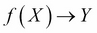The general structure of supervised learning algorithms is defined by the following decisions (Hand et al., 2001):

2. Decide on the machine learning algorithm, which introduces specific inductive bias, that is, apriori assumptions that it makes regarding the target concept.

3. Decide on the score or cost function, for instance, information gain, root mean square error, and so on.

4. Decide on the optimization/search method to optimize the score function.

5. Find a function that describes the relation between X and Y.

Many decisions are already made for us by the type of the task and dataset that we have. In the following sections, we will take a closer look at the classification and regression methods and the corresponding score functions.

### Classification

Classification can be applied when we deal with a discrete class, and the goal is to predict one of the mutually-exclusive values in the target variable. An example would be credit scoring, where the final prediction is whether the person is credit liable or not. The most popular algorithms include decision tree, naïve Bayes classifier, support vector machines, neural networks, and ensemble methods.

#### Decision tree learning

Decision tree learning builds a classification tree, where each node corresponds to one of the attributes, edges correspond to a possible value (or intervals) of the attribute from which the node originates, and each leaf corresponds to a class label. A decision tree can be used to visually and explicitly represent the prediction model, which makes it a very transparent (white box) classifier. Notable algorithms are ID3 and C4.5, although many alternative implementations and improvements (for example, J48 in Weka) exist.

#### Probabilistic classifiers

Given a set of attribute values, a probabilistic classifier is able to predict a distribution over a set of classes, rather than an exact class. This can be used as a degree of certainty, that is, how sure the classifier is in its prediction. The most basic classifier is naïve Bayes, which happens to be the optimal classifier if, and only if, the attributes are conditionally independent. Unfortunately, this is extremely rare in practice.

There is really an enormous subfield denoted as probabilistic graphical models, comprising of hundreds of algorithms; for example, Bayesian network, dynamic Bayesian networks, hidden Markov models, and conditional random fields that can handle not only specific relationships between attributes, but also temporal dependencies. Karkera (2014) wrote an excellent introductory book on this topic, Building Probabilistic Graphical Models with Python, while Koller and Friedman (2009) published a comprehensive theory bible, Probabilistic Graphical Models.

#### Kernel methods

Any linear model can be turned into a non-linear model by applying the kernel trick to the model—replacing its features (predictors) by a kernel function. In other words, the kernel implicitly transforms our dataset into higher dimensions. The kernel trick leverages the fact that it is often easier to separate the instances in more dimensions. Algorithms capable of operating with kernels include the kernel perceptron, Support Vector Machines (SVM), Gaussian processes, PCA, canonical correlation analysis, ridge regression, spectral clustering, linear adaptive filters, and many others.

#### Artificial neural networks

Artificial neural networks are inspired by the structure of biological neural networks and are capable of machine learning, as well as pattern recognition. They are commonly used for both regression and classification problems, comprising a wide variety of algorithms and variations for all manner of problem types. Some popular classification methods are perceptron, restricted Boltzmann machine (RBM), and deep belief networks.

#### Ensemble learning

Ensemble methods compose of a set of diverse weaker models to obtain better predictive performance. The individual models are trained separately and their predictions are then combined in some way to make the overall prediction. Ensembles, hence, contain multiple ways of modeling the data, which hopefully leads to better results. This is a very powerful class of techniques, and as such, it is very popular; for instance, boosting, bagging, AdaBoost, and Random Forest. The main differences among them are the type of weak learners that are to be combined and the ways in which to combine them.

#### Evaluating classification

Is our classifier doing well? Is this better than the other one? In classification, we count how many times we classify something right and wrong. Suppose there are two possible classification labels—yes and no—then there are four possible outcomes, as shown in the next figure:

• True positive—hit: This indicates a yes instance correctly predicted as yes

• True negative—correct rejection: This indicates a no instance correctly predicted as no

• False positive—false alarm: This indicates a no instance predicted as yes

• False negative—miss: This indicates a yes instance predicted as no

 Predicted as positive? Yes No Really positive? Yes TP—true positive FN—false negative No FP—false positive TN—true negative

The basic two performance measures of a classifier are classification error and accuracy, as shown in the following image: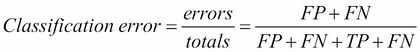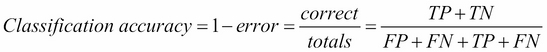The main problem with these two measures is that they cannot handle unbalanced classes. Classifying whether a credit card transaction is an abuse or not is an example of a problem with unbalanced classes, there are 99.99% normal transactions and just a tiny percentage of abuses. Classifier that says that every transaction is a normal one is 99.99% accurate, but we are mainly interested in those few classifications that occur very rarely.

##### Precision and recall

The solution is to use measures that don't involve TN (correct rejections). Two such measures are as follows:

• Precision: This is the proportion of positive examples correctly predicted as positive (TP) out of all examples predicted as positive (TP + FP):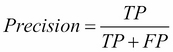• Recall: This is the proportion of positives examples correctly predicted as positive (TP) out of all positive examples (TP + FN):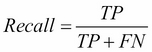It is common to combine the two and report the F-measure, which considers both precision and recall to calculate the score as a weighted average, where the score reaches its best value at 1 and worst at 0, as follows: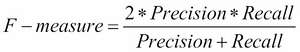##### Roc curves

Most classification algorithms return a classification confidence denoted as f(X), which is, in turn, used to calculate the prediction. Following the credit card abuse example, a rule might look similar to the following: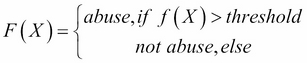The threshold determines the error rate and the true positive rate. The outcomes for all the possible threshold values can be plotted as a Receiver Operating Characteristics (ROC) as shown in the following diagram: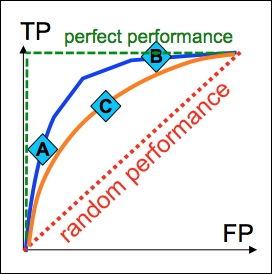A random predictor is plotted with a red dashed line and a perfect predictor is plotted with a green dashed line. To compare whether the A classifier is better than C, we compare the area under the curve.

Most of the toolboxes provide all of the previous measures out-of-the-box.

### Regression

Regression deals with continuous target variable, unlike classification, which works with a discrete target variable. For example, in order to forecast the outside temperature of the following few days, we will use regression; while classification will be used to predict whether it will rain or not. Generally speaking, regression is a process that estimates the relationship among features, that is, how varying a feature changes the target variable.

#### Linear regression

The most basic regression model assumes linear dependency between features and target variable. The model is often fitted using least squares approach, that is, the best model minimizes the squares of the errors. In many cases, linear regression is not able to model complex relations, for example, the next figure shows four different sets of points having the same linear regression line: the upper-left model captures the general trend and can be considered as a proper model, the bottom-left model fits points much better, except an outlier—this should be carefully checked—and the upper and lower-right side linear models completely miss the underlying structure of the data and cannot be considered as proper models.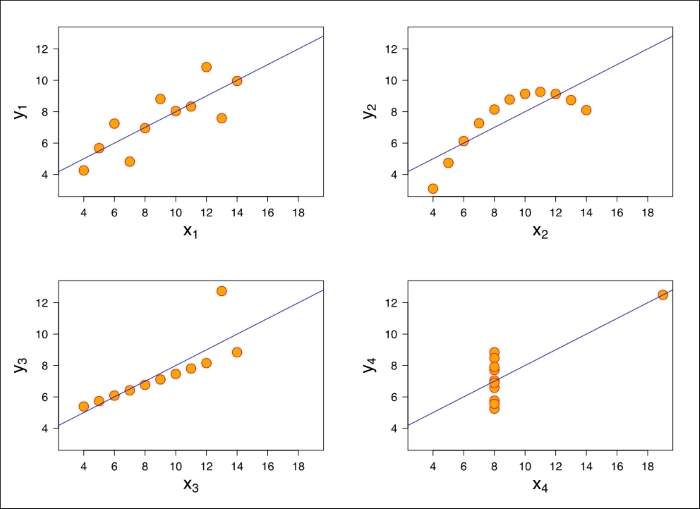#### Evaluating regression

In regression, we predict numbers Y from inputs X and the predictions are usually wrong and not exact. The main question that we ask is by how much? In other words, we want to measure the distance between the predicted and true values.

##### Mean squared error

Mean squared error is an average of the squared difference between the predicted and true values, as follows: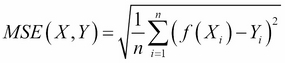The measure is very sensitive to the outliers, for example, 99 exact predictions and one predicton off by 10 is scored the same as all predictions wrong by 1. Moreover, the measure is sensitive to the mean. Therefore, relative squared error, which compares the MSE of our predictor to the MSE of the mean predictor (which always predicts the mean value) is often used instead.

##### Mean absolute error

Mean absolute error is an average of the absolute difference between the predicted and the true values, as follows: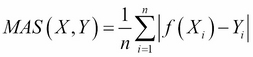The MAS is less sensitive to the outliers, but it is also sensitive to the mean and scale.

##### Correlation coefficient

Correlation coefficient compares the average of prediction relative to the mean multiplied by training values relative to the mean. If the number is negative, it means weak correlation, positive number means strong correlation, and zero means no correlation. The correlation between true values X and predictions Y is defined as follows: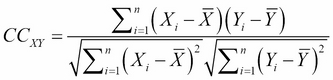The CC measure is completely insensitive to the mean and scale, and less sensitive to the outliers. It is able to capture the relative ordering, which makes it useful to rank the tasks such as document relevance and gene expression.

## Generalization and evaluation

Once the model is built, how do we know it will perform on new data? Is this model any good? To answer these questions, we'll first look into the model generalization and then, see how to get an estimate of the model performance on new data.

### Underfitting and overfitting

Predictor training can lead to models that are too complex or too simple. The model with low complexity (the leftmost models) can be as simple as predicting the most frequent or mean class value, while the model with high complexity (the rightmost models) can represent the training instances. Too rigid modes, which are shown on the left-hand side, cannot capture complex patterns; while too flexible models, shown on the right-hand side, fit to the noise in the training data. The main challenge is to select the appropriate learning algorithm and its parameters, so that the learned model will perform well on the new data (for example, the middle column):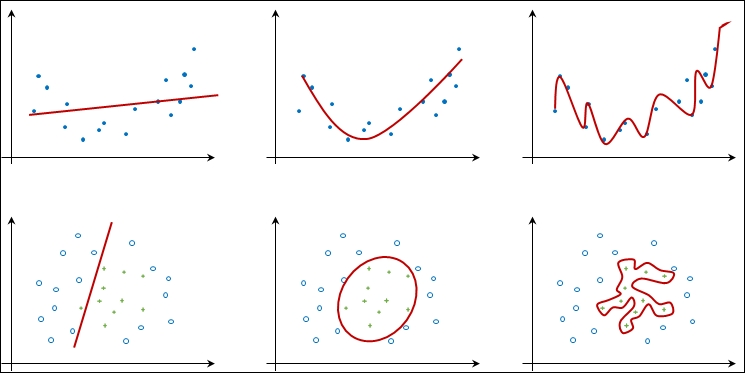The following figure shows how the error in the training set decreases with the model complexity. Simple rigid models underfit the data and have large errors. As model complexity increases, it describes the underlying structure of the training data better, and consequentially, the error decreases. If the model is too complex, it overfits the training data and its prediction error increases again: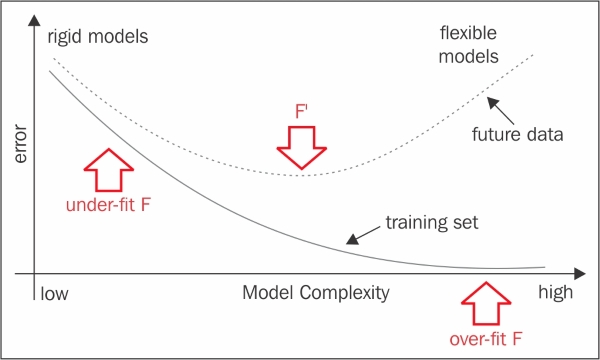Depending on the task complexity and data availability, we want to tune our classifiers towards less or more complex structures. Most learning algorithms allow such tuning, as follows:

• Regression: This is the order of the polynomial

• Naive Bayes: This is the number of the attributes

• Decision trees: This is the number of nodes in the tree, pruning confidence

• k-nearest neighbors: This is the number of neighbors, distance-based neighbor weights

• SVM: This is the kernel type, cost parameter

• Neural network: This is the number of neurons and hidden layers

With tuning, we want to minimize the generalization error, that is, how well the classifier performs on future data. Unfortunately, we can never compute the true generalization error; however, we can estimate it. Nevertheless, if the model performs well on the training data, but performance is much worse on the test data, the model most likely overfits.

#### Train and test sets

To estimate the generalization error, we split our data into two parts: training data and testing data. A general rule of thumb is to split them in the training:testing ratio, that is, 70:30. We first train the predictor on the training data, then predict the values for the test data, and finally, compute the error—the difference between the predicted and the true values. This gives us an estimate of the true generalization error.

The estimation is based on the following two assumptions: first, we assume that the test set is an unbiased sample from our dataset; and second, we assume that the actual new data will reassemble the distribution as our training and testing examples. The first assumption can be mitigated by cross-validation and stratification. Also, if it is scarce one can't afford to leave out a considerable amount of data for separate test set as learning algorithms do not perform well if they don't receive enough data. In such cases, cross-validation is used instead.

#### Cross-validation

Cross-validation splits the dataset into k sets of approximately the same size, for example, to five sets as shown in the following figure. First, we use the 2-5 sets for learning and set 1 for training. We then repeat the procedure five times, leaving out one set at a time for testing, and average the error over the five repetitions.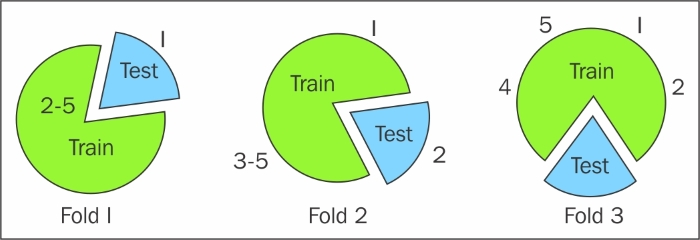This way, we used all the data for learning and testing as well, while we avoided using the same data to train and test a model.

#### Leave-one-out validation

An extreme example of cross-validation is the leave-one-out validation. In this case, the number of folds is equal to the number of instances; we learn on all but one instance, and then test the model on the omitted instance. We repeat this for all instances, so that each instance is used exactly once for the validation. This approach is recommended when we have a limited set of learning examples, for example, less than 50.

#### Stratification

Stratification is a procedure to select a subset of instances in such a way that each fold roughly contains the same proportion of class values. When a class is continuous, the folds are selected so that the mean response value is approximately equal in all the folds. Stratification can be applied along with cross-validation or separate training and test sets.

## Summary

In this chapter, we refreshed the machine learning basics. We revisited the workflow of applied machine learning and clarified the main tasks, methods, and algorithms. In the next chapter, we will review the kind of Java libraries that are available and the kind of tasks they can perform.